# Partial Fourier sum

A partial sum of the Fourier series of a given function.

In the classical one-dimensional case where a function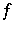is integrable on the segmentandis its trigonometric Fourier series, the partial Fourier sum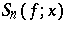of order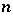ofis the trigonometric polynomial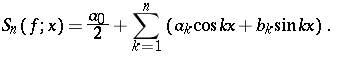With the use of the sequence of partial sums,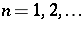, the notion of convergence of the seriesis introduced and its sum at a point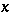is defined as follows: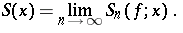At every point, the Dirichlet formula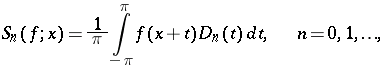is true; here,is the Dirichlet kernel of order. This formula plays a key role in many problems in the theory of Fourier series.

If a series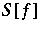is given in complex form, i.e., if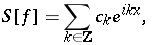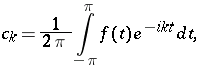where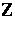is the set of all integers, thenIn the multi-dimensional case, a notion of partial sum can be introduced in numerous different ways, none of which can be regarded as preferable.

One of the possible general approaches is as follows: Let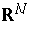be the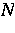-dimensional Euclidean space of points (vectors)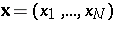, and let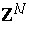be the integer lattice in, i.e., the set of vectorswith integer coordinates. For vectors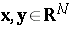, let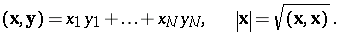Further, let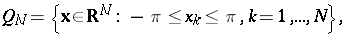let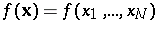be a function that is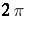-periodic in each variable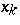and integrable over a cube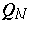, and let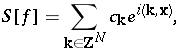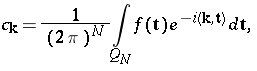be its Fourier series.

Further, let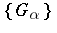be a family of bounded domains inthat depend on a real parameter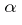and are such that any vector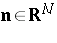belongs to all domains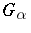for sufficiently large. In this case, the expressionis called a partial Fourier sum of the functioncorresponding to the domain, and the expression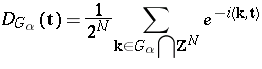is called the Dirichlet kernel corresponding to this domain. It is clear that, for any vector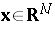, the following formula holds: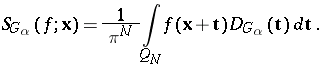This definition allows one to consider the problem of the convergence (or summability) of the seriesas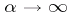. By virtue of the boundedness of the domains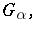the expression for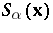is always a trigonometric polynomial.

The cases where-dimensional spheres or-dimensional intervals centred at the origin are taken asare most often encountered and are well studied. The expressions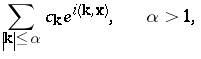are called spherical partial sums, and the expressionswhere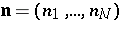is an arbitrary vector fromwith positive coordinates, are called rectangular partial sums. In recent years, in connection with problems in the approximation of functions from Sobolev spaces, partial Fourier sums constructed by "hyperbolic crosses" , namely, expressions of the form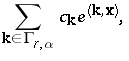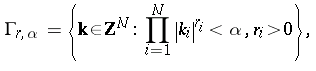have been extensively used. For Fourier series in general orthonormal systems of functions, partial Fourier series are constructed analogously. (Cf. also Orthonormal system.)

Various properties of partial Fourier sums and their applications to the theory of approximation and other fields of science can be found in, e.g., [a1], [a3], [a4], [a5], [a7]. [a6], [a2],